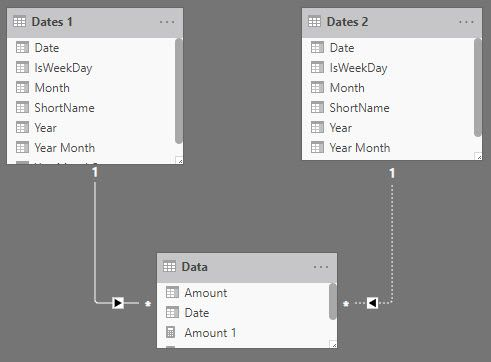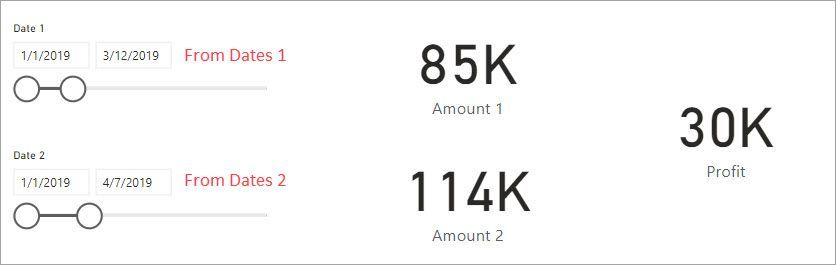cancel
Showing results for
Did you mean:Anonymous
Not applicable

## Calculating the difference between dates in the same column

Hi All,

I am new to power BI.

To explain my request is very simple (Dataset:financial data set from microsoft)

1. Create 2 date slicer based on the same "Date" column.
2. Display the profits based on the dates slicers selected
3. Calculate the difference of profit based on the dates selected and display it in a cardI have attached the visualization below1 ACCEPTED SOLUTIONSuper User

Hello @Anonymous

We jsut need a couple date tables, one inactive relationship and the right measure.Notice that the relationship between Dates 2 in the data is inactive.  We will turn it on in our mesure when needed.  We can get a simple date table with this DAX

```Dates 1 =
VAR DateRange = CALENDARAUTO()

RETURN
DateRange,
"Year",YEAR([Date]),
"Month",FORMAT([Date],"mmmm"),
"Year Month", FORMAT([Date],"yyyy-mmmm"),
"YearMonthSort",YEAR([Date])*100 + MONTH([Date]),
"ShortName",FORMAT([Date],"ddd"),
"IsWeekDay", NOT WEEKDAY( [Date] ) IN {1,7}
)```

Dates 2 is just this.

`Dates 2 = 'Dates 1'`

Amount 1 is straight sum using the first date relationship.

`Amount 1 = SUM ( Data[Amount] )`

Amount 2 is where we turn off the relationship with the first date table and turn on the link with the second one.

```Amount 2 =
CALCULATE(
SUM ( Data[Amount] ),
CROSSFILTER ( Data[Date], 'Dates 1'[Date], None), // Turns off the link to Dates 1
USERELATIONSHIP ( Data[Date], 'Dates 2'[Date] ) // Turns on the link to Dates 2
)```

Then the profit measure

`Profit = [Amount 2] - [Amount 1]`

And we get out desired result.I have attached my sample workbook for you to look at.

5 REPLIES 5Super User

Hello @Anonymous

We jsut need a couple date tables, one inactive relationship and the right measure.Notice that the relationship between Dates 2 in the data is inactive.  We will turn it on in our mesure when needed.  We can get a simple date table with this DAX

```Dates 1 =
VAR DateRange = CALENDARAUTO()

RETURN
DateRange,
"Year",YEAR([Date]),
"Month",FORMAT([Date],"mmmm"),
"Year Month", FORMAT([Date],"yyyy-mmmm"),
"YearMonthSort",YEAR([Date])*100 + MONTH([Date]),
"ShortName",FORMAT([Date],"ddd"),
"IsWeekDay", NOT WEEKDAY( [Date] ) IN {1,7}
)```

Dates 2 is just this.

`Dates 2 = 'Dates 1'`

Amount 1 is straight sum using the first date relationship.

`Amount 1 = SUM ( Data[Amount] )`

Amount 2 is where we turn off the relationship with the first date table and turn on the link with the second one.

```Amount 2 =
CALCULATE(
SUM ( Data[Amount] ),
CROSSFILTER ( Data[Date], 'Dates 1'[Date], None), // Turns off the link to Dates 1
USERELATIONSHIP ( Data[Date], 'Dates 2'[Date] ) // Turns on the link to Dates 2
)```

Then the profit measure

`Profit = [Amount 2] - [Amount 1]`

And we get out desired result.I have attached my sample workbook for you to look at.Anonymous
Not applicable

Thanks a lot @jdbuchanan71 @Anonymous  both the Methods are working.Anonymous
Not applicable

Hi,

I dont understand the reason to have two filters on the same Column. If you are looking to have two filters into the same Page then I would suggest you to do the below,

Make a copy of the Table the table with the required columns and write a measure that would say

SUM(TableCopy1[Profit])- SUM(TableCopy2[Profit]). Basically, you need to have the second Date filter coming from the Second table.Anonymous
Not applicable

@Anonymous  Thank you for the suggestion .. I already tried this method tried duplicating the table and used the one date from new source It worked fine.

Is there any othey way to do it other than duplicating the table?Anonymous
Not applicable

Hi,

I am doubtful if it is possible. I would however provide you with another suggestion.

Create a new Date Table that would contain all the distinct Values of the Dates from the first Table using the below formula.

`DATE:= DISTINCT(TableA[Date]) `

Once the Date Table is Created from the first Table, make sure there is no relationship between the TableA and the Date Table.

`VAR First_Date= FIRSTDATE(DATE[Date]) VAR Last_Date=LASTDATE(DATE[Date]) RETURN SUM(TableA[Profit])- SUMX(FILTER(TableA , TableA[Date]>=First_Date && TableA[Date]<= Last_Date),TableA[Profit])`